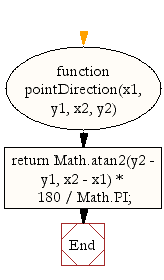# JavaScript: Calculate degrees between 2 points with inverse Y axis

## JavaScript Math: Exercise-27 with Solution

Write a JavaScript function to calculate degrees between 2 points with the inverse Y axis.

Test Data :
console.log(pointDirection(1, 0, 12, 0));
0
console.log(pointDirection(1, 0, 1, 10));
90

Sample Solution:-

HTML Code:

``````<!DOCTYPE html>
<html>
<meta charset="utf-8">
<title>Write a JavaScript function to calculate degrees between 2 points with inverse Y axis</title>
<body>

</body>
</html>
```
```

JavaScript Code:

``````function pointDirection(x1, y1, x2, y2) {
return Math.atan2(y2 - y1, x2 - x1) * 180 / Math.PI;
}

console.log(pointDirection(1, 0, 12, 0));
console.log(pointDirection(1, 0, 1, 10));
```
```

Sample Output:

```0
90
```

Flowchart:Live Demo:

See the Pen javascript-math-exercise-27 by w3resource (@w3resource) on CodePen.

Improve this sample solution and post your code through Disqus

What is the difficulty level of this exercise?

Test your Programming skills with w3resource's quiz.

﻿